Quantum Chemistry / DFT
Full Atomistic MD
Optical / Electrical / Magnetic
Materials Science

# Evaluation of Relative Permittivity Using MD and MO/DFT

Estimation of the relative permittivity of various molecules

## Purpose and method

There are three components of permittivity: electronic polarization, ionic polarization, and orientation polarization. In experiments, the sum of these is found as the permittivity, but when evaluated in simulations, the appropriate method must be selected and calculations performed for each.

### Evaluation by Molecular Dynamics (MD)

MD allows us to evaluate the polarization due to vibrations and orientations of molecules. The relative permittivity is obtained from the time fluctuations of the sum of the dipole moments obtained from the charges of each atom using the following formula;• µi : Dipole moment of molecule i
• qk : Atomic charge
• rk : Atomic position
• M : Sum of the molecular dipole moments
• εr: Relative Permittivity
• T: Temperature of the system
• V: Volume of the system

### Evaluation by Molecular Orbital method (MO) / Density Functional Theory (DFT)

MO/DFT allows us to evaluate the electron polarization. The relative permittivity is calculated from the molecular polarizability 𝛼 using the Clausius-Mossotti formula. The number density N must be obtained using experimental values, simulations, or QSPR.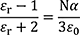• εr : Relative Permittivity
• ε0 : Permittivity of vacuum
• N : The number density of electric dipoles
• α : Polarizability

## Simulation results

Figure 2 and Table 1 show the results of the relative permittivity of benzene and acetone evaluated using MO and MD. The estimation values by using MO is obtained from the molecular polarizabilities determined by Gaussian09 and the experimental densities. In addition, the values by using MD is calculated from the liquid state bulk models at 300 K and 1 bar with OPLS force field. The εMD of acetone was also reported to be 15 for the same OPLS force field . So the J-OCTA calculation is also valid.

Since benzene has high symmetry and almost no permanent dipole, the relative permittivity using MD, which evaluates the polarization due to the vibrations and the orientations of the molecules, is very small. This indicates that most of the experimental values are due to electronic polarization. On the other hand, for acetone, it is not enough just to evaluate the electronic polarization, but it is important to evaluate the orientation polarization.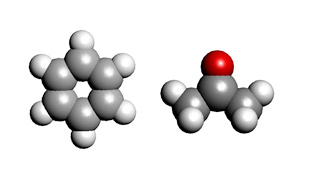Figure 1 The simulation models （Left : benzene、Right : acetone）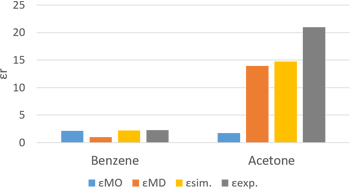Figure 2 The result of relative permittivity

Table 1 The results of relative permittivity and dipole moment

εMO εMD εsim. εexp.
Benzene 2.16 1.03 2.19 2.28
Acetone 1.76 13.96 14.72 21.01
Relative permittivity
μMO[D] μMD[D]
Benzene 0.013 0.190
Acetone 3.196 3.110
dipole moment

In addition, MD and Quantitative Structure Property Relationship (QSPR) were used to calculate the relative permittivity of the PVC polymers. The results are shown in Tables 2 and 3.
For MD, we created a PVC model with 10 repetitions and set up OPLS force field. 100 molecules were put into the system and relaxed at 300 K and 1 bar, and the relative permittivity was calculated from the MD results at 2 ns in equilibrium. The density at equilibrium was 1.32 g/cm3 and the relative permittivity determined by the MD was 2.92.
When estimating the relative permittivity by QSPR. The density of 1.38 g/cm3 and the relative permittivity of 2.93 are close to those of MD.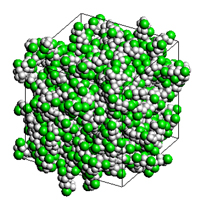Figure 3 The simulation model of PVC
（White: hydrogen, gray: carbon, green: chlorine ）

Table 2 The result of relative permittivity by MD
ρMD[g/cm3] εMD[-] µMD[D]
1.32 2.92 2.01
Table 3　The result of relative permittivity by QSPR
ρMD[g/cm3] ε298K[-]
1.3835 2.9289
• Reference
•  J.Bicerano, Prediction of Polymer Properties, 3rd Ed. Marcel Dekker, 2002
•  J. Chem. Eng. Data 2018, 63, 5, 1170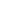This website uses cookies to improve functionality and performance. If you continue browsing the site, you are giving implied consent to the use of cookies on this website. If you want to know more or refuse consent, read our Cookie Policy.

Accept